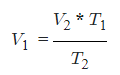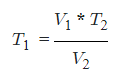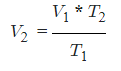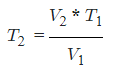Request a Tool

Charles Law Calculator

To calculate Initial Volume, final colume and Initial temperture and final temperture quantities in the Charles law of ideal gas

Output

Initial Volume (V1)
0

Output

Initial Temperature (T1)
0

Output

Final Volume (V2)
0

Output

Final Temperature
0

Formula• V1 = Initial Volume
• T1 = Initial Temperature
• V2 = Final Volume
• T2 = Final Temperature

Defination / Uses

Charles's Law is an ideal gas law where at constant pressure the volume of an ideal gas is directly proportional to its absolute temperature.

Uses

Real-life examples of the law used is Hot air balloon, Human lungs, Pool floats, Baking, Tyres and Helium balloon

Formula• V1 = Initial Volume
• T1 = Initial Temperature
• V2 = Final Volume
• T2 = Final Temperature

Defination / Uses

Charles's Law is an ideal gas law where at constant pressure the volume of an ideal gas is directly proportional to its absolute temperature.

Uses

Real-life examples of the law used is Hot air balloon, Human lungs, Pool floats, Baking, Tyres and Helium balloon

Formula• V1 = Initial Volume
• T1 = Initial Temperature
• V2 = Final Volume
• T2 = Final Temperature

Defination / Uses

Charles's Law is an ideal gas law where at constant pressure the volume of an ideal gas is directly proportional to its absolute temperature.

Uses

Real-life examples of the law used is Hot air balloon, Human lungs, Pool floats, Baking, Tyres and Helium balloon

Formula• V1 = Initial Volume
• T1 = Initial Temperature
• V2 = Final Volume
• T2 = Final Temperature

Defination / Uses

Charles's Law is an ideal gas law where at constant pressure the volume of an ideal gas is directly proportional to its absolute temperature.

Uses

Real-life examples of the law used is Hot air balloon, Human lungs, Pool floats, Baking, Tyres and Helium balloon

V1 and V2 be volume at temperature T1 and T2 such that pressure and amount of gas is constant. V1, T1 is volume and temperature at condition 1, and V2, T2 is volume and temperature at condition 2.
Establish the relationship between two equation V1/T1 = k and V2/T2= k. If the volume and temperature for a condition are known, we may use the following method to determine an unknown volume or temperature. Use the upper given formula for manual calculations. No sign-up, registration OR captcha is required to use this tool.